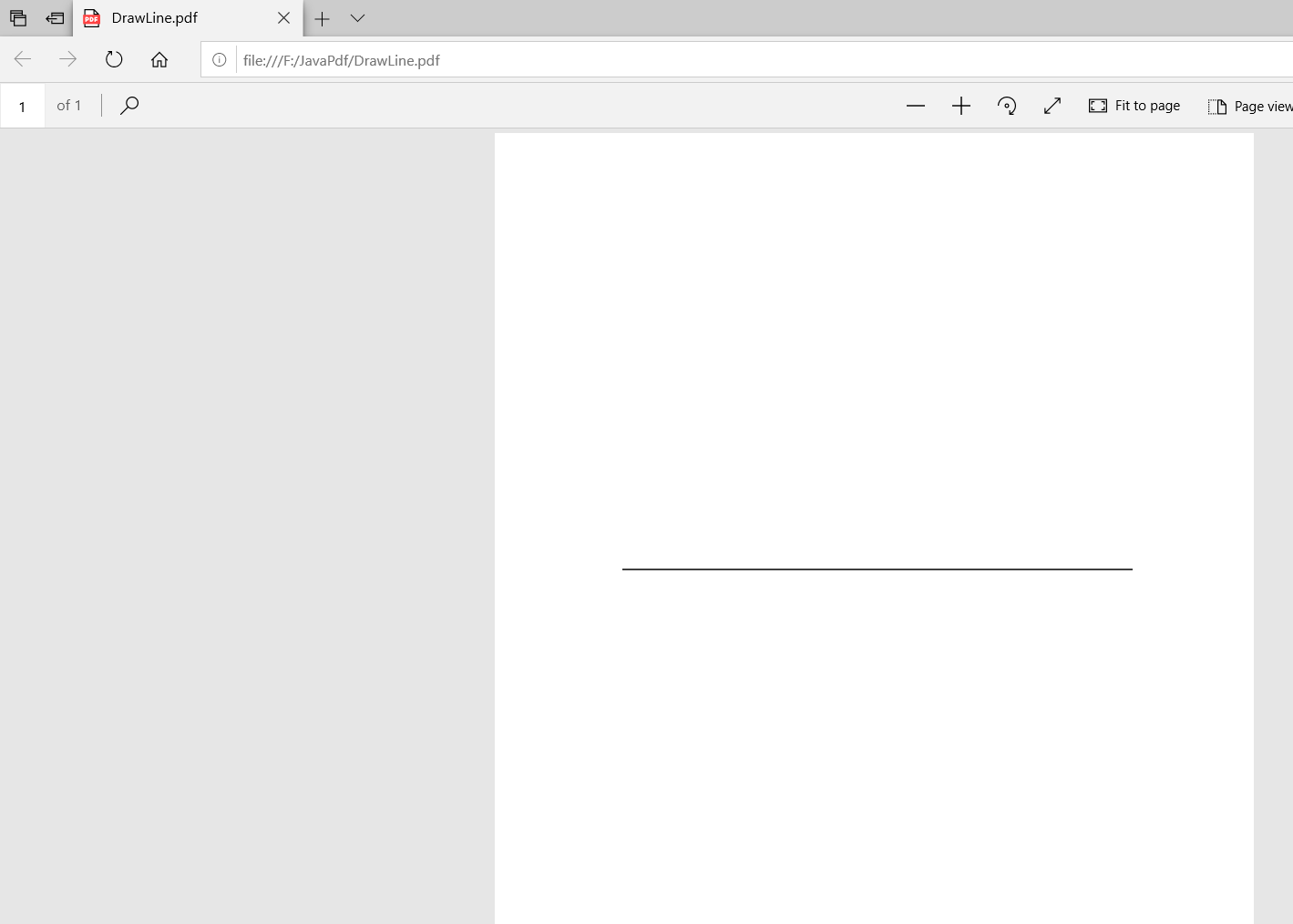Open in App
Not now

# Drawing a Line in a PDF Document using Java

• Last Updated : 17 Jun, 2021

In this article, we will learn how to Draw a line in a PDF document using Java. For drawing a line in a PDF, we will use the iText library. These are the steps that should be followed to Draw a line in a PDF using java.

1. Creating a PdfWriter object

The PdfWriter class represents the DocWriter for a PDF. The constructor of this class accepts a string, i.e. the path of the file where the PDF is to be created.

```// importing the PdfWriter class.
import com.itextpdf.kernel.pdf.PdfWriter;

// path where the pdf is to be created.
String path = "F:/JavaPdf/DrawLine.pdf";
PdfWriter pdfwriter = new PdfWriter(path);```

2. Creating a PdfDocument object

The PdfDocument class is that the class that represents the PDF Document in iText, To instantiate this class in write mode, you would like to pass an object of the category PdfWriter (i.e. pdfwriter from above code) to its constructor.

```// Creating a PdfDocument  object.
// passing PdfWriter object constructor of pdfDocument.
PdfDocument pdfdocument = new PdfDocument(pdfwriter); ```

3. Creating the Document object

The Document class is the root element when creating a self-sufficient PDF. One of the constructors of this class accepts an object of the type PdfDocument class(i.e. pdfdocument).

```// Creating a Document and passing pdfDocument object
Document document = new Document(pdfdocument);```

4.  Creating a PdfCanvas object

Before Instantiate the PdfCanvas object, We have to create a new pdfPage object as we need to pass pdfPage object to the constructor of PdfCanvas class.

```// Creating a new page

// instantiating a PdfCanvas object
PdfCanvas canvas = new PdfCanvas(pdfPage); ```

5.Drawing the line and closing the path stroke, document.

Set the starting point of the line using the moveTO() method of the Canvas class and  draw till the endpoint using the lineTo() method of the Canvas class.

```// starting point of the line
canvas.moveTo(100, 300);

// Drawing the line till the end point.
canvas.lineTo(500, 300);

// Close the path stroke
canvas.closePathStroke();

// Close the document
document.close(); ```

Dependencies for executing the below program:

```kernel-7.1.13.jar

layout-7.1.13.jar```

Example: Code to draw a line in a PDF document using java.

## Java

 `import` `com.itextpdf.kernel.pdf.PdfDocument;``import` `com.itextpdf.kernel.pdf.PdfPage;``import` `com.itextpdf.kernel.pdf.PdfWriter;``import` `com.itextpdf.kernel.pdf.canvas.PdfCanvas;``import` `com.itextpdf.layout.Document;` `public` `class` `DrawLine {``    ``public` `static` `void` `main(String args[]) ``throws` `Exception``    ``{``        ``try` `{``            ``// path where the pdf is to be created.``            ``String path = ``"F:/JavaPdf/DrawLine.pdf"``;``            ``PdfWriter pdfwriter = ``new` `PdfWriter(path);` `            ``// Creating a PdfDocument object.``            ``// passing PdfWriter object constructor of``            ``// pdfDocument.``            ``PdfDocument pdfdocument``                ``= ``new` `PdfDocument(pdfwriter);` `            ``// Creating a Document and passing pdfDocument``            ``// object``            ``Document document = ``new` `Document(pdfdocument);` `            ``// Creating a new page``            ``PdfPage pdfPage = pdfdocument.addNewPage();` `            ``// instantiating a PdfCanvas object``            ``PdfCanvas canvas = ``new` `PdfCanvas(pdfPage);` `            ``// starting point of the line``            ``canvas.moveTo(``100``, ``500``);` `            ``// Drawing the line till the end point.``            ``canvas.lineTo(``500``, ``500``);``          ` `              ``// close the path stroke``            ``canvas.closePathStroke();` `            ``// Close the document``            ``document.close();``            ``System.out.println(``                ``"drawn the line successfully"``);``        ``}``        ``catch` `(Exception e) {``            ``System.out.println(``                ``"Failed to draw the line due to "` `+ e);``        ``}``    ``}``}`

Compile and execute the program using the following commands

```javac RotateImage.java
java RotateImage```

Output

`drawn the line successfully`

PDFMy Personal Notes arrow_drop_up